##### Actions

The module of homomorphisms of a given module into the ground ring. More precisely, letbe a left module over a ring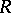. The Abelian group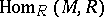of homomorphisms ofintoregarded as a left-module can be made into a right-moduleby putting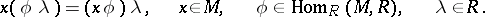This right moduleis called the adjoint of. For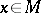, one can define an elementby puttingfor all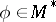. This defines a homomorphism ofinto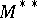. For any left-module, the mapping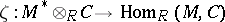given by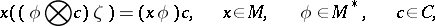is also a homomorphism. Both of these are isomorphisms whenis a finitely-generated projective module . It follows from the properties of the functor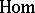that(where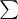is the direct sum, andthe direct product) and that there is a homomorphism of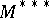into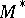. The composite mapping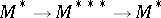is the identity, butneed not be isomorphic to. The torsion-free modules in the sense of Bass are those for which the above homomorphism ofintoturns out to be a monomorphism. This property is equivalent to the imbeddability ofin a direct product of copies of the ground ring. Ifis right and left Noetherian, then the mapping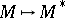defines a duality between the categories of finitely-generated left and right-modules if and only ifis a Quasi-Frobenius ring.